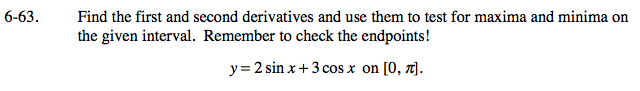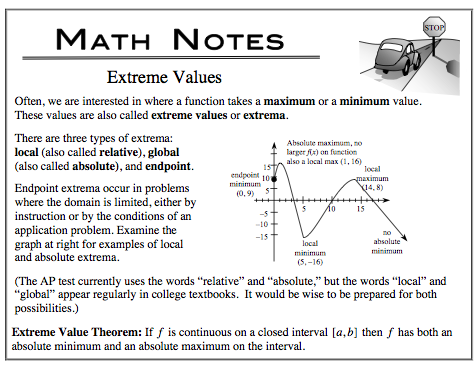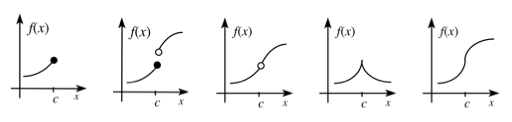### Home > CALC > Chapter 6 > Lesson 6.2.1 > Problem6-63

6-63.This problem is asking you to find EXTREME VALUES on a closed domain.
Extreme values (global maxima and global minima) can exist where y' = 0 or where y' = DNE.Find extrema candidates where y' = 0. Remember to only consider candidates within the given domain: [0, π].
y' = 2cosx − 2sinx = 2(cosx − sinx)
0 = 2(cosx − sinx)
[show steps]

Find more extrema candidates where y' = DNE. In other words, where is y non-differentiable? Are there endpoints, jumps, holes, cusps or vertical tangents? If so, these are extrema candidates. There are endpoint candidates at x = 0 and x = π.3 extrema candidates have been identified.

Test each candidate to see who has the most extreme (highest and lowest) y-values.
The 'winners' are the global max and the global min.

y(0) = _________

$y\left (\frac{\pi}{4} \right )=\underline{\ \ \ \ \ \ \ \ \ \ \ \ \ }$

y(π) = _________